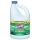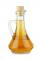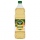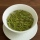# Clogging

How much distilled water must the pharmacist add to 30g of a 30% hydrogen peroxide solution to obtain a 3% solution to clogging?

Result

m =  270 g

#### Solution:

0.30 * 30 = 0.03 * (30+m)

0.03m = 8.1

m = 270

Calculated by our simple equation calculator.

Leave us a comment of example and its solution (i.e. if it is still somewhat unclear...):

Showing 0 comments:Be the first to comment!#### To solve this example are needed these knowledge from mathematics:

Our percentage calculator will help you quickly calculate various typical tasks with percentages. Do you have a linear equation or system of equations and looking for its solution? Or do you have quadratic equation? Tip: Our volume units converter will help you with converion of volume units.

## Next similar examples:

1. Alcohol mixingHow much 55% alcohol we must pour into 1500 g 80% alcohol to form a 60% alcohol? How much 60% alcohol created?
2. Home cleaningMr. Smith is cleaning up a big mess at home. In the closet, he finds a solution that is 5% bleach, and another stronger solution that is 20% bleach. For this particular job, he needs 600mL of 15% bleach. How much of each type (to the nearest mL) should he.
3. PineHow much of a mixed forest rangers want to cut down, if their head said: "We only cut down pine trees, which in our mixed forest 98%. After cut pine trees constitute 94% of all trees left."
4. Commission 2Mr gomez sells used cellphones. His commission for every cellphone sold is 20%. If his total sales is Php 33850, how much is his commission? Please, please, please show your solution.
5. Alcohol mixtureThree liters of 96 percent alcohol we set up a certain amount of distilled water to form a 54-percent alcohol. How many liters of distilled water were used?
6. Distilled waterSea water contains 5% salt. How many distilled water should be pour to 40 kg of sea water so that the salt content is 2%. How many kilograms of 2% of sea water we get?
7. ConcentrationHow 0.5 liters of 8% vinegar diluted to a concentration of 20 hundredths % of vinegar? How many liters of water must be pour?
8. SaltSea water containing 5% salt. How many kg of fresh water necessary to pour into 40 kg of sea water so that the salinity fell to 2%?
9. Acid evaporationHow many kilograms of water do we have to evaporate from 100 kg of 32% acid to make it 80% concentration?
10. Vinegar mixingWe mix 70g water and 50g 8% vinegar. How many percents of the solution of vinegar we produce?
11. Profit gainIf 5% more is gained by selling an article for Rs. 350 than by selling it for Rs. 340, the cost of the article is:
12. MushroomsMushrooms lose 90% by weight drying. How many fresh mushrooms are needed for 5 kg of dried mushrooms?
13. Theorem proveWe want to prove the sentense: If the natural number n is divisible by six, then n is divisible by three. From what assumption we started?
14. ConcentrationHow many grams of 65% and 50% acid is to be mixed to give 240 g acid with a concentration of 60%?
15. Acid solutionBy adding 250 grams of a 96% solution of sulfuric acid to its 3% solution its initial concentration was changed to 25%. How many grams of 3% of the acid were used for dilution?
16. Company and employeesThere are 370 employees in the company - women are 15% less than men. How many men work in the company?
17. Fruit teaTea contains 7% of fruit components and 12% of sugar in this component. How many percents of sugar is represented in the whole tea?